# On the map

A line 1.5 cm long corresponds to a line 3 cm long on the map. What is the scale of the map?

M =  50000

### Step-by-step explanation: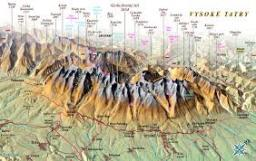Did you find an error or inaccuracy? Feel free to write us. Thank you!Tips to related online calculators
Do you want to convert length units?

## Related math problems and questions:

• Map 2At what scale is made map if the distance 8.2 km corresponds on the map segment 5 cm long?
• Map scale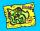What is the scale of the map, if 5.4 km is 72 mm on the map?
• Map scaleThe rectangular plot has a scale of 1: 10000 area 3 cm2 on the map. What content does this plot have on a 1:5000 scale map?
• Scale of the map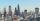The distance between two cities is actually 30 km and the map is 6 cm. What is the scale of the map?
• What scale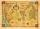What scale is the map drawn if it shows a 15km route from the station to the ruins with a line 30cm long? A) 1: 2000 B) 1: 5000 C) 1: 20,000 D) 1: 50,000
• The scaleThe distance of 15 cm on the map corresponds to a distance of 27 km in reality. What is the scale of the map?
• Scale of the mapDetermine the map's scale, which is the actual distance of 120 km l represented by a segment long 6 cm.
• Two citiesOn a map, 1.5 cm represents 50 miles. If the distance between the two cities on the map is 6 cm, how many miles separate the cities?
• The sidewalk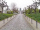The sidewalk is 50 m long. What will be its length on the plot plan on a scale of 1: 200?
• Plan scale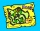The actual width of the room is 66 dm, the width in plan is 4.4 cm. What scale corresponds to the plan?
• PlotThe plot on which Mr. Kalous is to build a house has the shape of a rectangle. On a 1: 500 scale, its dimensions are 7cm and 5.5cm. Find out the dimensions of the plot. Calculate the parcel size.
• Map scaleGarden has on plan on a scale of 1: 150 width 22 cm and length 35 cm. What is the real area of the garden?
• Two villages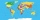Two villages are 11 km and 500 m away. On the map, their distance is determined by a 5 cm long line. Find the scale of the map.
• Small city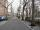The length of the street is 230 m. What will be the length of its image on the plan at a scale of 1: 2500?
• Scale 3Miriam room is 3.2 meters wide. It is draw by line segment length 6.4 cm on floor plan. In what scale it is plan of the room?
• The plan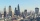The plan of the housing estate is in three scales 1: 5000,1: 10000,1: 15000. The distance between two points on a plan with a scale of 1: 10000 is 12 cm. What is this distance on the other two plans? What is this distance?
• Nautical miles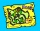How many nautical miles do they sail if the route is shown on a 1:25 000 scale map with a 7.4 cm long line?